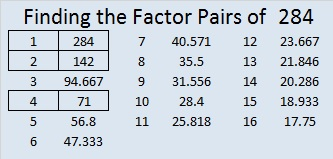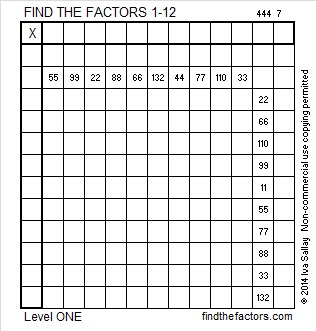# 284 and Level 1

• 284 is a composite number.
• Prime factorization: 284 = 2 x 2 x 71, which can be written 284 = 2² x 71
• The exponents in the prime factorization are 2 and 1. Adding one to each and multiplying we get (2 + 1)(1 + 1) = 3 x 2  = 6. Therefore 284 has exactly 6 factors.
• Factors of 284: 1, 2, 4, 71, 142, 284
• Factor pairs: 284 = 1 x 284, 2 x 142, or 4 x 71
• Taking the factor pair with the largest square number factor, we get √284 = (√4)(√71) = 2√71 ≈ 16.852Print the puzzles or type the factors on this excel file: 12 Factors 2014-11-03